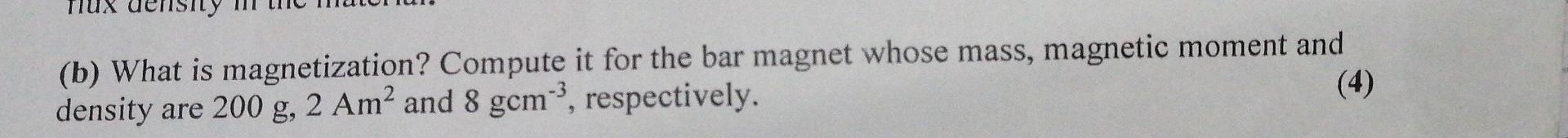# (Solved):   (b) What is magnetization? Compute it for the bar magnet whose mass, magnetic moment and d ...(b) What is magnetization? Compute it for the bar magnet whose mass, magnetic moment and density are $$200 \mathrm{~g}, 2 \mathrm{Am}^{2}$$ and $$8 \mathrm{gcm}^{-3}$$, respectively.

We have an Answer from Expert